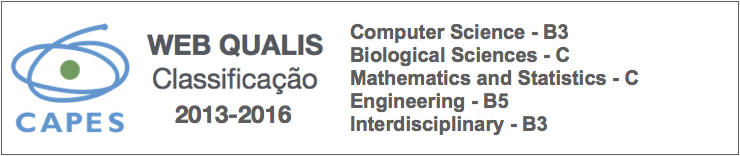### Derivation of Mean Value Coordinates Using Interior Distance and Their Application on Mesh Deformation

Lis Custódio, Sinesio Pesco

#### Abstract

The deformation methods based on cage controls became a subject of considerable interest due its simplicity and intuitive results. In this technique, the model is enclosed within a simpler mesh (the cage) and its points are expressed as function of the cage elements. Then, by manipulating the cage, the respective deformation is obtained on the model in its interior.

In this direction, in the last years, extensions of barycentric coordinates, such as Mean Value coordinates, Positive Mean Value Coordinates, Harmonic coordinates and Green's coordinates, have been proposed to write the points of the model as a function of the cage elements.

The Mean Value coordinates, proposed by Floater in two dimensions and extended later to three dimensions by Ju et al. and also by Floater, stands out from the other coordinates because of their simple derivation. However the existence of negative coordinates in regions bounded by non-convex cage control results in a unexpected behavior of the deformation in some regions of the model.

In this work, we propose a modification in the derivation of Mean Value Coordinates proposed by Floater. Our derivation maintains the simplicity of the construction of the coordinates and eliminates the undesired behavior in the deformation by diminishing the negative influence of a control vertex on regions of
the model not related to it. We also compare the deformation generated with our coordinates and the deformations obtained with the original Mean Value coordinates and Harmonic coordinates.

Computer Science

PDF

#### References

SEDERBERG, T. W.; PARRY, S. R. Free-form deformation of solid geometric models. SIGGRAPH Comput. Graph., v. 20, n. 4, p. 151–160, 1986.

COQUILLART, S. Extended free-form deformation: a sculpturing tool for 3d geometric modeling. SIGGRAPH Comput. Graph., v. 24, n. 4, p. 187–196, 1990.

KOBAYASHI, K. G.; OOTSUBO, K. t-ffd: free-form deformation by using triangular mesh. In: TURKIYYAH,

G.; BRUNET, P. (Ed.). Proceedings of the eighth ACM symposium on Solid modeling and applications. Seattle, WA, USA: ACM, 2003. (SM ’03, v. 1).

FLOATER, M. S. Mean value coordinates. Comput Aided Geom Des, v. 20, n. 1, p. 19–27, 2003.

JU, T.; SCHAEFER, S.; WARREN, J. Mean value coordinates for closed triangular meshes. ACM Trans Graph., v. 24, n. 3, p. 561–566, 2005.

FLOATER, M. S.; KOS, G.; REIMERS, M. Mean value coordinates in 3d. Comput Aided Geom Des, v. 22, n. 7, p. 623–631, 2005.

LI, X.-Y.; JU, T.; HU, S.-M. Cubic mean value coordinates. ACM Trans Graph., v. 32, n. 4, p. 126:1–10, 2013.

RUSTAMOV, R. M. Barycentric coordinates on surfaces. Comput. Graphs. Forum, v. 29, n. 5, p. 1507–1516, 2010.

FARBMAN, Z. et al. Coordinates for instant image cloning. ACM Trans. Graph., v. 28, n. 3, p. 67:1–9, 2009.

JOSHI, P. et al. Harmonic coordinates for character articulation. ACM Trans Graph., v. 26, n. 3, p. 71:1–71:10, 2007.

LIPMAN, Y. et al. Gpu-assisted positive mean value coordinates for mesh deformations. In: SGP ’07. Proceedings of the Fifth Eurographics Symposium on Geometry Processing. Aire-la-Ville, Switzerland: Eurographics Association, 2007. v. 1, p. 117–123.

LIPMAN, Y.; LEVIN, D.; COHEN-OR, D. Green coordinates. ACM Trans. Graph., v. 27, n. 3, p. 78:1–78:10, 2008.

SCHAEFER, J. M. andScott. Moving least squares coordinates. Comput. Graphs. Forum, v. 29, n. 5, p. 1517–1524, 2010.

CUSTODIO, L. Extenso ̃es de Coordenadas Bariceˆntricas para Deformac ̧a ̃o de Malhas. Dissertac ̧a ̃o (Mestrado) — Departamento de Matema ́tica, PUC-Rio, Rio de janeiro, Brazil, 2010.

RUSTAMOV, R. M.; LIPMAN, Y.; FUNKHOUSER, T. Interior distance using barycentric coordinates. In: Proceedings of the Symposium on Geometry Processing. Aire-la-Ville, Switzerland: Eurographics Association, 2009. (SGP ’09, v. 1), p. 1279–1288.

JU, T.; LIEPA, P.; WARREN, J. A general geometric construction of coordinates in a convex simplicial polytope. Comput Aided Geom Des, v. 24, n. 3, p. 161–178, 2007.

DOI: https://doi.org/10.22456/2175-2745.76189

Copyright (c) 2018 Lis Custodio, Sinesio Pesco